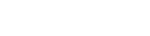# Extracting DC Network Nodes

Since I implemented the loop optimizing code, I realized that I needed to extract nodes that form a network for DC analysis before generating the loops for this.

So I deleted the code that I wrote previously to convert loops to those for DC Analysis which was very simple. It’s actually more complicated when you consider a bunch of interconnected inductors, since we need to merge the nodes that they interconnect with.

I purposely created some unlikely circuits such as inductors in series to test this out.

Here is the code that I came up with:

``````func get_dc_net_nodes(net_nodes_):
var regex = RegEx.new()
regex.compile("^L\\d*")
var nodes = []
# Remove capacitors
for node in net_nodes_:
for pin in node:
if pin.begins_with("ECap") or pin.begins_with("C"):
node.erase(pin)
# Combine inductor nodes
var inductor_nodes = {}
for node in net_nodes_:
var no_inductor = true
for pin in node:
var result = regex.search(pin)
if result:
if inductor_nodes.keys().has(pin):
inductor_nodes[pin].append_array(node)
else:
inductor_nodes[pin] = node
no_inductor = false
if no_inductor and node.size() > 0:
nodes.append(node)
# Combine common nodes resulting from the previous operations
for key in inductor_nodes.keys():
for k2 in inductor_nodes.keys():
if k2 != key:
for pin in inductor_nodes[k2]:
if pin == key:
inductor_nodes[k2].append_array(inductor_nodes[key])
inductor_nodes[key].clear() # Don't replicate these pins
# Remove inductor pins
for key in inductor_nodes.keys():
var pins_to_erase = []
for k2 in inductor_nodes.keys():
for pin in inductor_nodes[key]:
if pin == k2:
pins_to_erase.append(pin)
for pin in pins_to_erase:
inductor_nodes[key].erase(pin)
for node in inductor_nodes.values():
if node.size() > 0:
nodes.append(node)
return nodes
``````

This was tough to code! One of the weird bugs, which is due to a common mistake was where I was deleting elements of an array that was being traversed. You can see where I added `pins_to_erase` to overcome this problem.

It reduces the original network of nodes down to a network of nodes that applies to the DC state of the circuit. Then we may run this through the loop extraction functions as usual.

Also, now I have made the code open source here: Electronics Simulation with GDScript

## More Devlog entries

Most recent first